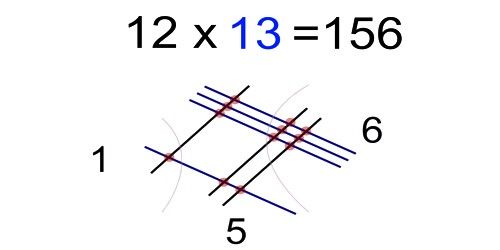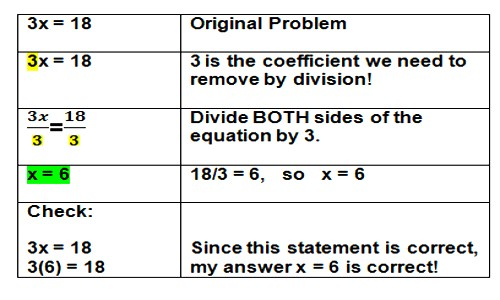Mathematic

# Multiplication EquationsMultiplication Equations

To solve a multiplication equation, use the inverse operation of division. Divide both sides by the same non-zero number.

An equation is a mathematical statement such that the expressions on the left side of the equals sign (=) has the same value as the expression on the right side. An example of an equation is 9 * 8 = 72.

One of the terms in an equation may not be known and needs to be determined. The unknown term may be represented by a letter such as x. (e.g. x * 8 = 72).

The solution of an equation is finding the value of the unknown x. Use the division property of equations to find the value of x. The division property of equations states that the two sides of an equation remain equal if both sides are divided by the same number

Example:

x * 5 = 10

x * 5 ÷ 5 = 10 ÷ 5

x * 1 = 2

x = 2

Check the answer by substituting the answer (2) back into the equation.

2 * 5 = 10An equation is a mathematical statement such that the expression on the left side of the equals sign (=) has the same value as the expression on the right side. An example of an equation is 12 * 11 = 132.

One of the terms in an equation may not be known and needs to be determined. The unknown term may be represented by a letter such as x. (e.g. x * 11 = 132).

The solution of an equation is finding the value of the unknown x. Use the division property of equations to find the value of x. The division property of equations states that the two sides of an equation remain equal if both sides are divided by the same number

Example:

x * 50 = 1000

x * 50 ÷ 50 = 1000 ÷ 50

x * 1 = 20

x = 20

Check the answer by substituting the answer (20) back into the equation.

20 * 50 = 1000.

Information Source: